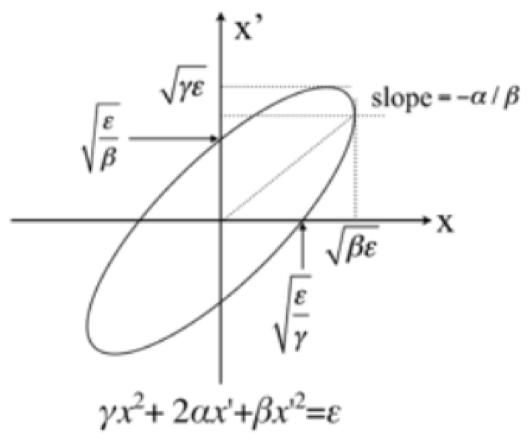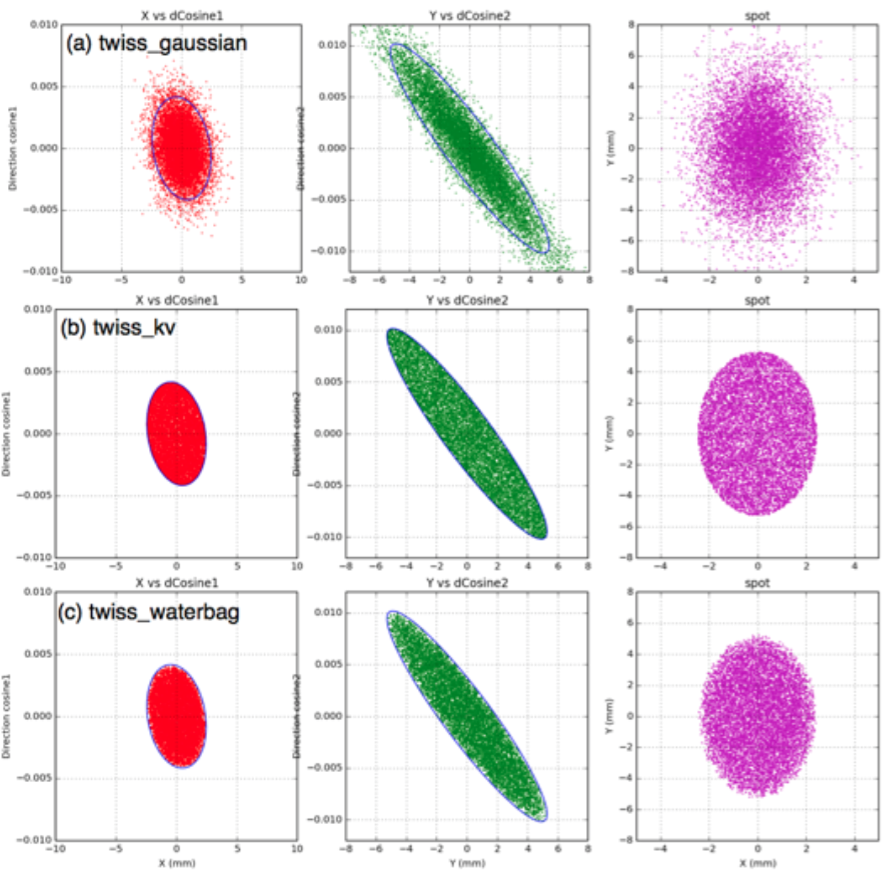# Emittance Sources¶

Emittance sources provide 4 ways of sampling particles’ position (X and Y) and momentum direction (X’ and Y’) on a plane. Specify source type as:

```s:So/MySource/Type = "emittance"
```

To sample particle position and momentum direction from “Gaussian” (bivariate 2D gaussian) per axis (see the Emittance_Gaussian.txt example):

```s:So/MySource/Distribution = "BiGaussian" # distribution name
d:So/MySource/SigmaX = 0.2 mm # std of x positions
u:So/MySource/SigmaXprime = 0.032 # std of x’, note that it’s unitless. 1 equals to 1.0 rad.
u:So/MySource/CorrelationX = -0.9411 # correlation of x and x’
d:So/MySource/SigmaY = 0.2 mm # std of y positions
u:So/MySource/SigmaYPrime = 0.032 # std of y’
u:So/MySource/CorrelationY = 0.9411 # correlation of y and y’
```

Alternative distributions are TWISS Gaussian, TWISS Kapchinskij-Vladimirskij (KV), and TWISS Waterbag (based on the Courant-Snyder invariant ellipse in the following figure for X axis only):Additional parameters specify the TWISS alpha, beta and gamma as shown in the following from Emittance_Twiss.txt:

```s:So/MySource/Distribution = "twiss_gaussian" # "twiss_gaussian", "twiss_kv" or "twiss_waterbag"
u:So/MySource/AlphaX = 0.2
d:So/MySource/BetaX  = 600.0 mm
d:So/MySource/EmittanceX = 0.01 mm # we don’t multiply pi intrinsically.
u:So/MySource/AlphaY = 2.5
d:So/MySource/BetaY = 1400.0 mm
d:So/MySource/EmittanceY = 0.02 mm
# below for twiss gaussian option only
#0.9 means that 90 % particles will be included in ellipse circle
u:So/MySource/ParticleFractionX = 0.90
u:So/MySource/ParticleFractionY = 0.90
```

The energies and species of the emitted particles and the Cutoff Shape Parameters can be specified using the same parameters available to the Beam Sources.

The following images based on the Emittance_Twiss.txt example show how particle position and momentum directions are sampled and the shape of the beam spots (purple). The blue solid lines in X (red dots) and Y (green dots) represent the Courant-Snyder invariant ellipse. For example, 90% of particles are sampled from the given emittance ellipse in (a).